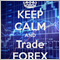# Filter Hours of trading - page 2115

serpentsnoir & bkpleng pointed to it, but I didn't see it.

//NOTE the origial code had return(0); if((Hour()<=StartTime || Hour()>EndTime))return(0); //Preferd Trading Hours

Which worked great when trading from 6-16

These are the ones that seems to work when looking to trade from 6-10 & 13-16

if((Hour()<=0 && Hour()>=5) || (Hour()<=11 && Hour()>=12) || (Hour()<=17 && Hour()>=23))return(0);

if(Hour()==6 || Hour()==7 || Hour()==8 || Hour()==9 || Hour()==10 || Hour()==13 || Hour()==14 || Hour()==15 || Hour()==16)

if((Hour()>=6 && Hour()<=10) || (Hour()>=13 && Hour()<=16))

Thank you all for your help.17401

What value of Hour would make this true ? (Hour()<=0 && Hour()>=5)25364

I_Need_Money:

From an older EA, which I believed tested successfully but is not working.
if((Hour()<=7 || Hour()>=10) || (Hour()<=14 || Hour()>=18))return(0); //Prefer Trading Hours

hour 0 .. 7 (true || false) || (true || false) = (true) || (true) = true = return

hour 8, 9 (false || false) || (true || false) = (false) || (true) = true = return

hour 10 .. 14 (false || true) || (true || false) = (true) || (true) = true = return

hour 15 .. 17 (false || true) || (false || false) = (true) || (false) = true = return

hour 18 .. 23 (false || true) || (false || true) = (true) || (true) = true = return

Instead, document what you actually mean, no thinking required:

```bool morningHours   = (Hour() >  7 && Hour() < 10),
afternoonHours =  Hour() > 14 && Hour() < 18,115

RaptorUK:
What value of Hour would make this true ? (Hour()<=0 && Hour()>=5)

This sample has "return(0); " so looking to trade hours not listed. So the EA will not trade during 00 same as 24 & 05:00 hours115

WHRoeder:

hour 0 .. 7 (true || false) || (true || false) = (true) || (true) = true = return

hour 8, 9 (false || false) || (true || false) = (false) || (true) = true = return

hour 10 .. 14 (false || true) || (true || false) = (true) || (true) = true = return

hour 15 .. 17 (false || true) || (false || false) = (true) || (false) = true = return

hour 18 .. 23 (false || true) || (false || true) = (true) || (true) = true = return

Instead, document what you actually mean, no thinking required:

```bool morningHours   = (Hour() >  7 && Hour() < 10),
afternoonHours =  Hour() > 14 && Hour() < 18,
Nice and very clean, also a fan of no thinking required.2397

I dont think that would work correctly.

 int Hour( )
Returns the hour (0,1,2,..23) of the last known server time by the moment of the program start (this value will not change within the time of the program execution).17401

I_Need_Money:

This sample has "return(0); " so looking to trade hours not listed. So the EA will not trade during 00 same as 24 & 05:00 hours
You didn't answer my question . . but never mind.[Deleted]
`This is my first attempt at coding a function to handle opening hours. it takes into account hours and minutes. If you find use for it, good!`
```extern bool UseTradingHours = true;
extern int OpenHour = 08;
extern int OpenMin = 30;
extern int CloseHour = 17;
extern int CloseMin = 30;

//-------------------------------------

{
execute();
}

//---------------------------------------

{
if(CloseHour>OpenHour) //within the day
{
if (OpenHour < TimeHour(TimeCurrent()) && TimeHour(TimeCurrent()) < CloseHour)
{
return(true);
}
if (OpenHour == TimeHour(TimeCurrent()))
{
if(OpenMin<=TimeMinute(TimeCurrent()))
{
return(true);
}
return(false);
}

if (CloseHour == TimeHour(TimeCurrent()))
{
if(CloseMin>=TimeMinute(TimeCurrent()))
{
return(true);
}
return(false);
}
Comment("Closed");
return(false);
}
if(OpenHour>CloseHour)  //Spanning two days
{
if (CloseHour < TimeHour(TimeCurrent()) && TimeHour(TimeCurrent()) < OpenHour)
{
Comment("Closed");
return(false);
}
if (OpenHour == TimeHour(TimeCurrent()))
{
if(OpenMin<=TimeMinute(TimeCurrent()))
{
return(true);
}
return(false);
}
if (CloseHour == TimeHour(TimeCurrent()))
{
if(CloseMin>=TimeMinute(TimeCurrent()))
{
return(true);
}
return(false);
}
return(true);
}
}```1924

hi there,

I'm keen to do this; but I would require:

Allow trades between the minutes of: 05->25 and 40->55

```bool firsthalf   = (Minute() >  5 && Minute() < 25),
secondhalf =  Minutes() > 35 && Minutes() < 55,

I imagine this would work?81

Chan Qing Huang:

Hello,

It is very good, but when you compile you have the "not all control paths return a value" warning.

After trying and looking in the forums (I'm a beginner in MQL4) I have found the solution:

I have changed  if(OpenHour>CloseHour)  //Spanning two days"

into else

Additionally, for better performance (with the same hour and different minutes, for example, 8:00 and 8:30)

I have change if(CloseHour>OpenHour) //within the day

into if(CloseHour>=OpenHour) //within the day

(I have added the symbol = ).